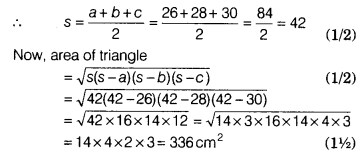# A reservoir is in the form of a rectangular parallelopiped (cuboid). Its length is 20 m

A reservoir is in the form of a rectangular parallelopiped (cuboid). Its length is 20 m. If 18 k1 of water is removed from the reservoir, the water level goes down by 15 cm. Find the width of the reservoir. [use 1 k1 =lm3]

Let sides of triangle be a = 26 cm, b = 28cm, c = 30 cm.∵ Area of parallelogram = Area of triangle (given)
⇒ b x h = 336 ⇒ 28 x h = 336
⇒ h=336/28=12 cm
Hence, the height of the parallelogram is 12 cm